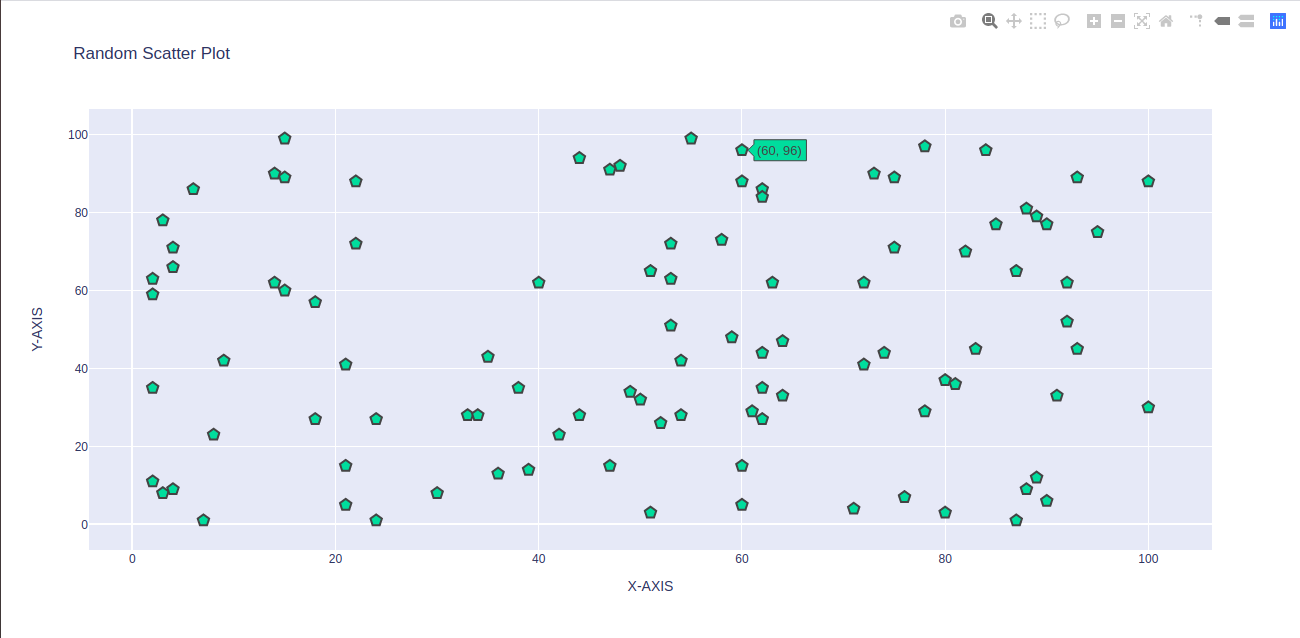# How to make a basic Scatterplot using Python-Plotly?

Plotly is a graphing library for making high quality interactive graphs and charts. It is an open source library used for data visualization . This library can be used with three programming languages namely Python, R and Javascript. Using Plotly is very easy and you can make any type of graph using this library.  It can generate Statistical charts, financial charts, Scientific charts, maps , 3D charts, subplots, etc.

But there are many other libraries like seaborn , matplotlib, bokeh available for data visualization. Then, why to choose Plotly?

• It provides large number of functions to visualize any kind of data .
• It is very simple, user friendly yet flexible.
• It allows you to embed your interactive plots in your project using HTML.
• Compatible with number of programming languages like ruby, python, javascript, matlab, etc.
• Plotly also have Plotly Chart Studio where you can directly edit your plots without writing single line of code.

## How to make a basic Scatterplot using Plotly and Python ?

Let’s start with making a very basic plot known as  Scatterplot. Scatterplot helps in finding the relationship between two variables . It tells us whether the two variables are positively related, negatively related or not related at all.

Example:

## Python3

 `import` `plotly.offline as pyo ` `import` `plotly.graph_objs as go ` `import` `numpy as np ` ` `  ` `  `# creating random data through randomint ` `# function of numpy.random ` `np.random.seed(``42``) ` ` `  `random_x``=` `np.random.randint(``1``,``101``,``100``) ` `random_y``=` `np.random.randint(``1``,``101``,``100``) ` ` `  `# create variable data which holds the data ` `data``=``[go.Scatter(x``=``random_x, ` `                 ``y``=``random_y, ` `                 ``mode``=``'markers'``, ` `                 ``marker``=` `dict``(size``=` `12``, ` `                               ``color``=` `'rgb(51,204,153)'``, ` `                               ``symbol``=` `'pentagon'``, ` `                               ``line``=` `{``'width'``:``2``} ` `                               ``) )] ` ` `  `# create layout of scatter plot ` `layout``=``go.Layout(title``=``'Random Scatter Plot'``, ` `                 ``xaxis``=` `{``'title'``:``'X-AXIS'``}  , ` `                 ``yaxis``=` `dict``(title``=``'Y-AXIS'``), ` `                 ``hovermode``=` `'closest'` `) ` ` `  `# create figure variable to pass the ` `# data and Layout ` `fig``=` `go.Figure(data``=``data , layout``=``layout) ` ` `  `# call plot function using plotly offline ` `pyo.plot(fig, filename``=``'scatterplot-1.html'``)`

Output :-Note: Last line of code will generate a file named scatterplot-1.html . Then open this file in your web browser . You will see the same output there.

My Personal Notes arrow_drop_upCheck out this Author's contributed articles.

If you like GeeksforGeeks and would like to contribute, you can also write an article using contribute.geeksforgeeks.org or mail your article to contribute@geeksforgeeks.org. See your article appearing on the GeeksforGeeks main page and help other Geeks.

Please Improve this article if you find anything incorrect by clicking on the "Improve Article" button below.

Article Tags :

1

Please write to us at contribute@geeksforgeeks.org to report any issue with the above content.